## Error Propagation

Given a Formula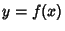with an Absolute Error inof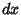, the Absolute Error is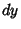. The Relative Error is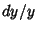. If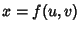, then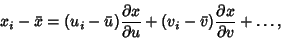(1)

so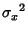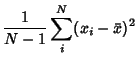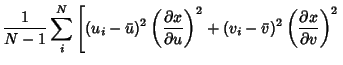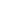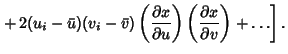(2)

The definitions of Variance and Covariance then give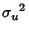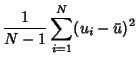(3)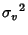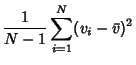(4)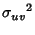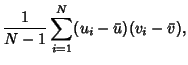(5)

so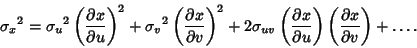(6)

If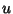and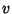are uncorrelated, then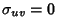so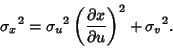(7)

Now consider addition of quantities with errors. For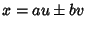,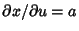and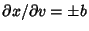, so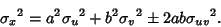(8)

For division of quantities with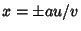,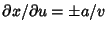and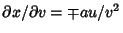, so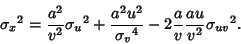(9)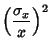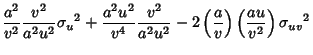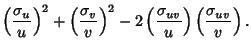(10)

For exponentiation of quantities with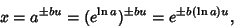(11)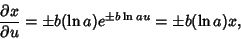(12)

so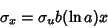(13)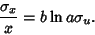(14)

If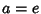, then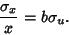(15)

For Logarithms of quantities with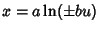,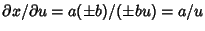, so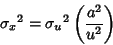(16)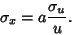(17)

For multiplication with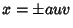,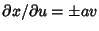and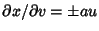, so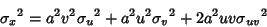(18)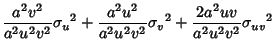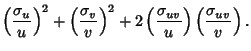(19)

For Powers, with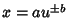,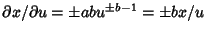, so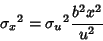(20)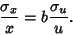(21)